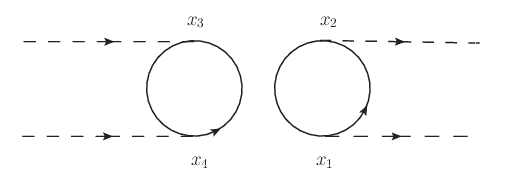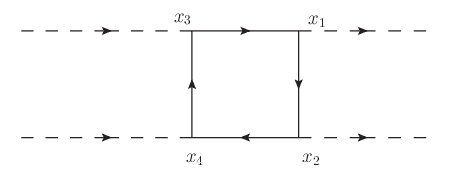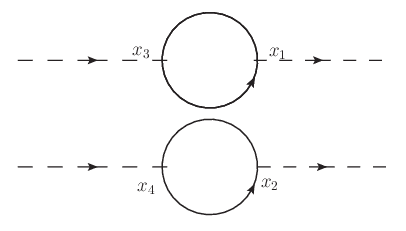# Scalar-scalar scattering Feynman diagram

• A
Gold Member
I'm trying to work out the Feynman diagrams for scalar-scalar scattering using the Yukawa interaction, as given in Chapter 6 of Lahiri & Pal's A First Book of Quantum Field Theory. The interaction hamiltonian is $$\mathscr{H}_{I}=h:\overline{\psi}\psi\phi:$$ where ##\psi## is a fermion field and ##\phi## is the scalar field. The scattering to be studied is ##BB\rightarrow BB##, where ##B## is a scalar (boson).
If I understand the procedure, we start with an initial state of two bosons (with given momenta) and end up with a final state of two bosons. Thus we must form products of ##\mathscr{H}_{I}## that contain two scalar annihilation operators on the right and two scalar creation operators on the left, which would require a product of 4 time-ordered terms:
$$\mathscr{T}\left[:\overline{\psi}\psi\phi:_{x_{1}}:\overline{\psi}\psi\phi:_{x_{2}}:\overline{\psi}\psi\phi:_{x_{3}}:\overline{\psi}\psi\phi:_{x_{4}}\right]$$
where the subscripts ##x_1## and so on indicate the spacetime coordinates of each term.
When expanding this using Wick's theorem, we would need to contract all pairs of operators involving ##\overline{\psi}## and ##\psi## (ignoring equal-time contractions). As far as I can tell, this gives rise to 2 types of terms (sorry, but I can't find a way of indicating the underlying brackets for contractions):
1. Contract ##\overline{\psi}\left(x_{1}\right)## with ##\psi\left(x_{2}\right)##, ##\psi\left(x_{1}\right)## with ##\overline{\psi}\left(x_{2}\right)##, ##\overline{\psi}\left(x_{3}\right)## with ##\psi\left(x_{4}\right)## and ##\psi\left(x_{3}\right)## with ##\overline{\psi}\left(x_{4}\right)##(and permutations of the spacetime coords)
2. Contract ##\overline{\psi}\left(x_{1}\right)## with ##\psi\left(x_{2}\right)##, ##\psi\left(x_{1}\right)## with ##\overline{\psi}\left(x_{3}\right)##, ##\overline{\psi}\left(x_{2}\right)## with ##\psi\left(x_{4}\right)## and ##\psi\left(x_{3}\right)## with ##\overline{\psi}\left(x_{4}\right)##(and permutations of the spacetime coords)
If those are correct, it would seem that the Feynman diagram of (1) is:and of (2) is:A couple of questions...
1. Is that correct?
2. If so, is the first diagram meaningful, seeing as it's disconnected so there doesn't seem to be any way of getting from the incoming particles to the outgoing ones?

#### Attachments

Gold Member
You're missing a few more disconnected diagrams. (I'll leave it to you to play around and find them, but you can ask if you still don't see them. EDIT: Actually, I missed that you specified permutations of the diagrams you've shown, so you're only missing one disconnected diagram + permutations of it.)

However, the disconnected diagrams do not contribute to physical processes due to the "cancellation of disconnected diagrams" which occurs when you normalize the correlation function by the vacuum amplitude (whose corrections must also be computed). In particular, the correlation function of the interacting theory is given by

$$\langle A B C \cdots \rangle = \frac{\langle A B C \cdots e^{- i \int dt H_I} \rangle_0}{\langle e^{- i \int dt H_I} \rangle_0}$$

where $\langle \rangle_0$ denotes an expectation value with respect to the non-interacting vacuum, $H_I$ is the interaction Hamiltonian in the interaction picture, and all expectation values are time-ordered. The numerator is the sum of all diagrams (disconnected or not), and the denominator needs to be computed by considering all digrams with no external legs ("vacuum bubbles"). Then the key result, which should be proven in every QFT textbook, is that when you expand the resulting ratio as a power series in the coupling, you get the same result that you would have by just considering connected diagrams.

It's a good exercise to check that this works out, so maybe you could try showing it explicitly in your case.

Last edited:
•Glenn Rowe
Gold Member
Thanks. I haven't got to the point in the book yet where I can actually do calculations with the S-matrix or Feynman diagrams so I'll leave those for a while.
At this stage, I'm just trying to ensure that I know how to generate the diagrams correctly, so it's reassuring to know I'm on the right track.

Gold Member
Aha - I think the remaining diagram looks like this:The point is that we have to contract the fields in pairs of spacetime points, otherwise we're left with a single time point that would need to contract with itself, and we're not allowed to do same-time contractions (according to Lahiri & Pal, anyway).

#### Attachments

Gold Member
Those diagrams were the ones which are permutations of the first diagram in your first post (e.g. just permuting external legs).

The remaining diagrams involve completely contracting the external $\phi$ fields and then the vertices can only contract with themselves. To steal an image I found on Google, I'm thinking of the image on the right here: https://www.colorado.edu/physics/phys7270/phys7270_fa16/images/bubbles-2pt.png except there is another disconnected line connecting 3 and 4. (Plus you should permute all possible ways of contracting the external lines.) The diagram on the left of the linked picture would not contribute in your case since you've normal-ordered the interaction.

Gold Member
I'm a bit confused now. I thought that if we had two incoming bosons (represented by ##\phi##) and two outgoing bosons, then we'd need the four ##\phi## fields to be uncontracted in all diagrams, since they are required to annihilate the incoming and create the outgoing particles. In other words, I thought that the only contractions we'd have to do would be all the ##\overline{\psi}## with the ##\psi## fields, since no fermions are involved in the incoming or outgoing states.

Gold Member
No, the relevant matrix element you need to compute is got four-scalar scattering is
$$\langle \phi(y_1) \phi(y_2) \phi(y_3) \phi(y_4) \rangle_{\mathrm{amp.},\mathrm{conn.}}$$
where "amp." means "amputated," meaning you leave out particular diagrams where external propagators have internal loops, and "conn." means only connected diagrams. This is the quantity that enters the LSZ formula for scalar-scalar scattering, which should be derived in your text.

I made a bit of a mistake above - the disconnected diagrams which contain vacuum bubbles are the ones which cancel out in the formula I gave above. In contrast, the diagrams like the one you gave in post #4 actually will contribute to the $\langle \phi \phi \phi \phi \rangle$ correlation function, but they are not included in computing scattering cross sections because they just contribute to the $\mathbb{I}$ part of the S matrix when written $S = \mathbb{I} - i T$ (they don't involve scattering). Meanwhile, diagrams like the first one in your post #1 are simply impossible due to momentum conservation.

The remaining diagram comes from evaluating
$$\langle \phi(y_1) \phi(y_2) \phi(y_3) \phi(y_4) \phi(x_1) \bar{\psi}(x_1) \psi(x_1) \phi(x_2) \bar{\psi}(x_2) \psi(x_2) \phi(x_3) \bar{\psi}(x_3) \psi(x_3) \phi(x_4) \bar{\psi}(x_4) \psi(x_4) \rangle_{\mathrm{amp.},\mathrm{conn.}}$$
(we don't even need the normal-ordering signs here because the "conn." makes this equivalent to the contribution with normal ordering.) Then by Wick's theorem, the non-zero contribution to this must involve contractions of the "external" $\phi$'s with the interaction vertices. So in this correlation function, you also get factors for the external propagators (but those factors are not used for computing the S matrix as per the LSZ formula).

What textbook are you using?

Gold Member
It looks like I need to read a fair bit further into the books to understand this. I don't see where the ##\phi(y_i)## terms come from - they appear to be extra terms beyond the Yukawa interaction? Maybe I just need to be patient and read a bit more...

I'm using Lahiri & Pal A First Book in Quantum Field Theory as my main source. I also look at Lancaster & Blundell's Quantum Field Theory for the Gifted Amateur and Greiner & Reinhardt's Field Quantization occasionally. I have tried Peskin & Schroeder, Zee and Srednicki but I find all 3 of these books impenetrable after the first few pages. Neither Lahiri & Pal nor Lancaster & Blundell mention LSZ (at least it's not in the index), though Greiner & Reinhardt do, but it's a fair ways into the book so I probably wouldn't be able to understand it yet.
I've read only up to the beginning of Chapter 6 in Lahiri & Pal, where they introduce Feynman diagrams and give an example of a boson decaying to two fermions using the Yukawa interaction. I haven't got to calculating matrix elements yet, though that's what they do next.